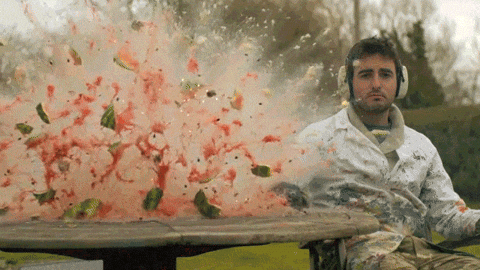# Explosions

We have found that can be achieved by collisions consisting of objects in contact being forced apart, similar to the way that pieces of an object separate during an explosion.  Explosions must also obey the . If an object starts at rest, then when we add up the momentum of every piece of the object after the explosion we must end up with zero, just as in the previous examples of collisions with only two “pieces”. Watching an explosion in reverse helps to illustrate this point:After the explosion of the watermelon the total momentum must be zero because it was zero before the explosion. The momentum of pieces moving in any one direction must cancel out the momentum of pieces moving in the opposite direction. Therefore, we see no particular direction that has noticeably more pieces, larger pieces, or faster moving pieces. The explosion is symmetric. (The table does redirect some pieces upward, so the explosion might not appear completely symmetric in the vertical direction.)

If we really pay attention to the watermelon explosion we can see that no pieces move downward because the table redirects them. If we were to actually add up all the momenta of all the pieces we would find that the watermelon system had a net upward . There was an external acting on the system of watermelon pieces and therefore the momentum of the watermelon system was not in this explosion. If we include the table and the earth it’s attached to in our system, then the forces between the watermelon pieces and the table are internal to our system and we would find that the momentum of that system was conserved. This idea is summarized by the , which states that whenever the net external force on a system is zero, the momentum of the system will not change. The is very similar and sort of tells us how to test the principle: If you include all of the objects involved in a collision, so that there are no external forces from objects outside the system, and you add up the momenta of all those objects before and then again after a collision you will get the same value.

# Impulse

When the net external force on a system is not zero, such as for the watermelon, then the momentum will change and the tells us how:

(1)We can see that the change in momentum is calculated as initial subtracted from final, similar to changes in velocity, position, time, or any other quantity. The right side of the equation, average force multiplied by the collision time, is known as the (I). The force and resulting impulse on the watermelon system from the table were upward, therefore the momentum of the watermelon system changed from zero to upward.

# Jet Propulsion

The flyboard in the previous video operates with an externally located pump that shoots water up the hose to the board which re-directs it downward. The of the water was not conserved when it collided with the board, therefore it must have felt an , a.k.a a net force for some amount of time. tells us that the board must have felt an equal and opposite force back during that time, and that force balances the of the board and rider.

### Everyday Examples

Let’s estimate the mass flow rate (mass of water expelled per time) required just to hold up the board and a person.  This video is helpful.

The board of the board and rider is 93 kg, so multiplying by g (9.8 m/s/s) we get a of 911 N. The board must apply  911 N of downward force on the water in order to receive that same size upward force. The on the water resulting from the downward force will change its according to the :Assuming the water moves up the hose, bounces off the board, and exits at the same speed it entered, then the initial water momentum is just the opposite (negative) of the final momentum:The double negative on the left becomes an addition:We don’t have a specific mass of water having a specific collision because the process appears continuous, but we could think image many tiny chunks of water colliding,  (even to the point of individual water molecules). We can we write the final of each water chunk in terms of its and final :Now if we divide each side by two and by the final velocity we can isolate the mass:We don’t know thefor each collision, but we don’t need to. Dividing by the time interval will give us the water mass that collides per time interval, which is exactly what we want:Running the video in slow motion I made a very rough estimate of the water exit and found 16 m/s downward. We already know the force on the water needs to be 911  N downward, so we can enter those values:The board must redirect 30 kg of water each second in order to hold the rider. Water has a of 1 Liter per kg, so 30 liters of water pass through the system each second. You may have noticed that “bouncing the water off the board, instead of just shooting it out like a rocket, reduced the flow rate by half. This is because this “bounce” doubled the on the water (and back on the board). We will talk more about bouncy collisions in the next chapter.

# Rocket Locomotion

Some machines actually use real chemical explosions for . For example, rocket fuel burns to produce hot, rapidly expanding gas which shoots out of the rocket backward, providing an on the rocket. The force associated with that impulse is known as thrust. The analysis we used for the fly board doesn’t really work for the rocket because the of the rocket changes as it burns fuel, but that analysis is very interesting and you should ask your instructor about it.